Machine_Parts_Index
Spring Index

Helical Spring surge/ Natural frequency

INTRODUCTION

If a spring which is subject to a vibratory motion which is close to its natural frequency the spring can start to surge.    This situation is very undesireable because the life of the spring can be reduced as excessive internal stresses can result.     The operating characteristics of the spring are also seriously affected.     For most springs subject to low frequency vibrations surging is not a problem.    However for high frequency vibrating applications it is necessary to to ensure, in the design stage, that the spring natural frequency is 15 to 20 or more times the maximum operating vibration frequency of the spring.

Nomenclature

 C = Spring Index D/d d = wire diameter (m) D = Spring diameter = (Di+Do)/2 (m) Di = Spring inside diameter (m) Do = Spring outside diameter (m) Dil = Spring inside diameter (loaded ) (m) E = Young's Modulus (N/m2) F = Axial Force (N) Fi = Initial Axial Force (N)      (close coiled tension spring) G = Modulus of Rigidity (N/m2) K d = Traverse Shear Factor = (C + 0,5)/C K W = Wahl Factor = [(4C-1)/(4C+5)}]+ (0,615/C) L = length (m) L 0 = Free Length (m) L s = Solid Length (m) k = Spring rate = M / δ (N/m.) M = mass supported by spring (kg) n t = Total number of coils na = Number of active coils p = pitch (m) u = motion of spring element(m) y = distance from neutral axis to outer fibre of wire (m) σ = Tensile/compressive stress (N/m2 ) ωn = Natural angular frequency of spring (rads/s) fn = Natural frequency of spring (cycles/s) τ = shear stress (N/m2) τ i = initial spring stress (N/m2) τ max = Max shear stress (N/m2) θ = Deflection (radians) δ = linear deflection (mm)

Note: metres (m) have been shown as the units of length in all of the variables above for consistency.   In most practical calculations milli-metres will be more convenient.

Spring Rate

Generally springs are designed to have a deflection proportional to the applied load (or torque -for torsion springs).   The "Spring Rate" is the Load per unit deflection.... Rate (N/mm) = F(N) / δ e(deflection=mm)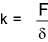Natural Frequency of a Loaded spring System

Consider a mass M supported on a weightless spring with a spring rate k is illustrated below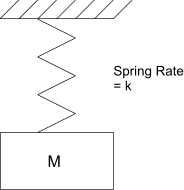This system has a natural frequency as shown below.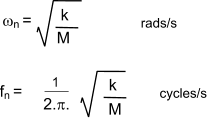This frequency is the lowest natural frequency and is the most important natural frequency.     The spring , however not weightless and thus it has vibration characteristics of its own.    Vibration effects within the spring and the associated frequencies are found by examinging a small element of the spring in harmonic motion.

Surge / Natural frequency of spring

For more detailed notes refer to webpage Simple Harmonic Motion

Consider a spring below subject to a vibration amplitude u at a circular frequency ω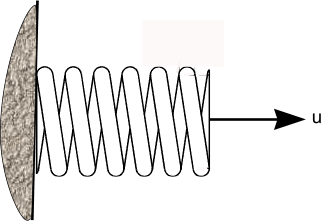Consider a small element of the spring comprising dn coils.     The density of the spring material is ρ and the length of the wire in the element is π.D.dn.     If the wire diameter is d then the element has a mass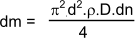For a vibration amplitude u at a circular frequency ω and at any axial location from coil n = 0 to coil n = na the inertial force amplitude is as follows.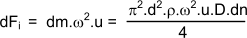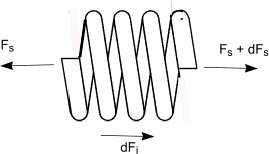The spring rate k for a helical spring is derived on webpage Helical Springs as ..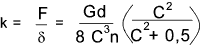In practice the term (1 + 0,5/C2) which approximates to 1 can be ignored

For the purpose of this analysis this is transformed to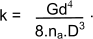This equation represents the force for na active coils .

The spring force amplitude per active coil resulting from a spring deflection per coil du/dn is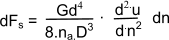Equating the forces on the spring element to zero the equation below is derived.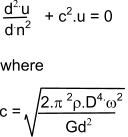This equation is solved using the equation.

u = A sin(c.n) + B cos(c.n)

A and B are arbitrary constants.
For a spring fixed at one end and free at the other the boundary condition occurs at

u = 0 occurs at coil 0
and the condition du/dn = 0 is applied at n = na

Resulting in the solution B = 0 and cos(c.na) = 0 from which

c.na = a.π / 2               ( a= 1,3,5 .....)

This results in a set of natural(surge)frequencies as follows..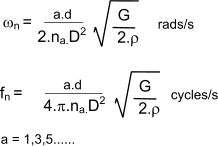For the important fundamental natural frequency, this can be simplified to..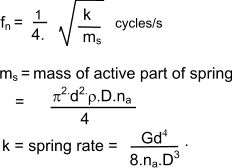Import Note : The above analysis relates to a spring with one end against a flat surface and the other end free.     The fixed.. fixed case i.e a spring located between and in full contact with two plates results in similar solutions where a = 2, 4, 6......This is also applicable to a spring with one end fixed and against a plate and the other end driven with a sin-wave motion.     The fundamental surge frequency resulting from these scenarios are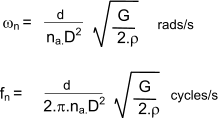Links to Spring Design efunda Notes on Natural frequency of springs Acxess Springs ...Information on Design, Materials etc (Imperial) Earthlink- How to Make Springs ...Good simple comprehensive information Lee Springs ...Spring Supplier + useful technical Information Springmasters ...Spring Supplier + comprehensive range of springs with sizes and ratings

Machine_Parts_Index
Spring Index